Time：2022-6-3

# brief introduction

This paper introduces the implementation of finite normal mixture model in R software for model-based clustering, classification and density estimation. The EM algorithm is used to estimate the parameters of normal mixed models with various covariance structures, and the simulation functions based on these models are provided. In addition, it also includes the function of combining model-based hierarchical clustering, EM of mixed distribution estimation and Bayesian information criterion (BIC), and a comprehensive strategy for clustering, density estimation and discriminant analysis. Other functions can be used to display and visualize fitting models as well as clustering, classification and density estimation results.

# clustering

``head(X)``
``pairs(X)````plot(BIC)````summary(BIC)````summary(mod1, parameters = TRUE)````plot(mod1)````table(class, classification)``
``plot(mod1, what = "uncertainty")````````clustICL(X)
summary(ICL)````````BootstrapLRT(X)``

# initialization

EM algorithm is used for maximum likelihood estimation. The initialization of EM is performed using partitions obtained from the cluster hierarchy clustering.

`` hclust(X, use = "SVD"))````Clustbic (x, initialization)) \``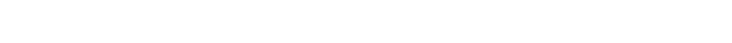``hc2````clustBIC(X, initialization )````hclust(X, model= "EEE"))````summary(BIC3)``Update BIC by merging best results.

``BIC(BIC1, BIC2, BIC3)``Univariate fitting using random starting points is obtained by creating random agglomerations and merging best results.

``````for(j in 1:20)
{
rBIC <- mclustBIC(
initi ))
BIC <- update(BIC, rBIC)
}````````clust(ga, BIC)``# classification

## EDDA

``````X <- iris\[,1:4\]``clustDA(X, class,  "EDDA")````plot(mod2)``## MclustDA

``table(class)````head(X)````clustDA(X, class)````plot(mod3, 2)````plot(mod3, 3)``# Cross validation error

``cv(mod2, nfold = 10)``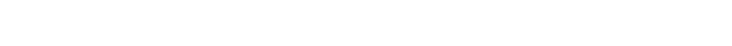``unlist(cv\[3:4\])``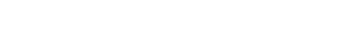``cv(mod3, nf = 10)``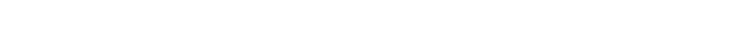``unlist(cv\[3:4\])``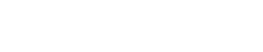# density estimation

## Univariate

``clust(acid)``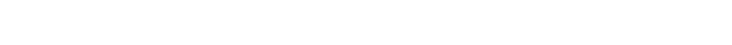``plot(mod4, "BIC")````plot(mod4,  "density", acidity)````plot(mod4, "diagnostic",  "cdf")``# Multivariable

``````clu(faithful)
summary(mod5)````````plot(mod5, "BIC")````````

plot(mod5, "density",faithful)``````# Bootstrap inference

``summary(boot1, what = "se")````summary(boot1, what = "ci")````````summary(boot4, what = "se")

plot(boot4)``````# Dimensionality reduction

## clustering

``plot(mod1dr,  "pairs")````plot(mod1dr)````plot(mod1dr, "scatterplot")````plot(mod1dr)``## classification

``````summary(mod2dr)

plot(mod2d)````````plot(mod2dr)````````summary(mod3dr)

plot(mod3dr)````````plot(mod3dr)``# Using the palette

Most drawings use the default color.

The palette can be defined and assigned to the above options as follows.

``````options("Colors" = Palette )
Pairs(iris\[,-5\], Species)``````If desired, users can easily define their own palettes.

# reference

Fraley C. and Raftery A. E. (2002) Model-based clustering, discriminant analysis and density estimation, _Journal of the American Statistical Association_, 97/458, pp. 611-631.Most popular insights

2.Case implementation of panel smooth transition regression (PSTR) analysisAnalysis case implementation “)

## Records about the common problems of Microsoft office 2021 home and student versions _ the shadow of excel in the cell selection is stuck and delayed during the process of pulling down the data area and is out of sync with the mouse pointer!

The problem has been tested on the home and student versions of office 2021 on 2 computers, and the same problem occurs The mouse operation is to pull down at a constant speed. Pay attention to the change speed of the number of lines. The number of pull-down lines in the data area changes slowly […]